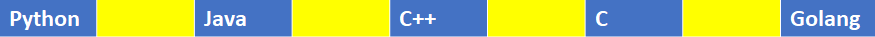# Python | Split String and Keep Whitespace

5/5 - (1 vote)

Summary: To split a string and keep the delimiters/separators, you can use one of the following methods: (i) Using the regex package and its functions. (ii) Using a list comprehension.

### Minimal Example

```import re
text = "Python  Java  C++  C  Golang"

# Method 1
print(re.split(r'(\s+)', text))

# Method 2
print(re.split('([^a-zA-Z0-9+]+)', text))

# Method 3
res = re.compile(r'(\S+)').split(text)
print([x for x in res if x != ''])

# Method 4
res = [u for x in text.split('  ') for u in (x, '  ')]
res.pop()
print(res)```

## Problem Formulation

Problem: Given a string in Python. How to split the string and also keep the spaces?

### Example

Consider that there’s a given string as shown in this example below and you need to split it such that the spaces present between the words are also stored along with the word characters in a list. Please follow the example given below to get an overview of our problem statement.

```# Input
text = "Python  Java  C++  C  Golang"
# Output
['Python', '  ', 'Java', '  ', 'C++', '  ', 'C', '  ', 'Golang']```

Graphical Illustration of the problem:fig: The Blue Boxes represent the word characters/strings while the Yellow Boxes represent the spaces in between the words.

Now that we have an overview of our problem, let us dive into the solutions without any delay!

## Method 1: Use Regular Expressions(RegEx)

### Method 1.1: Using re.split

One of the ways in which we can split the given string along with the spaces is to import the regex module and then split the string using the` re.split()` function and passing a special pattern within it as shown in the solution below.

```import re

text = "Python  Java  C++  C  Golang"
print(re.split(r'(\s+)', text))```

Output

`['Python', '  ', 'Java', '  ', 'C++', '  ', 'C', '  ', 'Golang']`

Let us examine and discuss the expression used here:

• `\s+` is a special sequence that returns a match where it does not find any word characters in the given string. Here it is used to find the spaces while splitting the string.
• `()` is used to ensure that the separators/delimiters (in this case space) along with the word characters are considered and preserved in the resultant list.

### Method 1.2: Using [^]

Another way of splitting the string using regex is to split it using the `split()` function along with the `([^a-zA-Z0-9]+)` as the pattern within it. Let’s have a look at the code and then we will dive deep into the pattern used here.

Code:

```import re

text = "Python  Java  C++  C  Golang"
print(re.split('([^a-zA-Z0-9+]+)', text))```

Output

`['Python', '  ', 'Java', '  ', 'C++', '  ', 'C', '  ', 'Golang']`

Let us examine the expression used here:

• `()` ensures that the spaces (i.e. the delimiter) are preserved while splitting the string.
• `[]` is used to match a set of characters within the string.
• `[^a-zA-Z0-9+]+` is used to return a match for any character EXCEPT alphabets (both Capital Letters and Small Letters), Numbers and a `+` sign i.e. it is simply used to find spaces which is the delimiter/separator in this case.

### Method 1.3: Use re.compile and split

Approach: Use the compile method of the regex library to split at non whitespace characters.

Code:

```import re
text = "Python  Java  C++  C  Golang"
res = re.compile(r'(\S+)').split(text)
print([x for x in res if x != ''])```

Output

`['Python', '  ', 'Java', '  ', 'C++', '  ', 'C', '  ', 'Golang']`

Note: The method `re.compile(pattern)` returns a regular expression object from the `pattern` that provides basic regex methods such as `pattern.search(string)``pattern.match(string)`, and `pattern.findall(string)`. The explicit two-step approach of (1) compiling and (2) searching the pattern is more efficient than calling, say, `search(pattern, string)` at once, if you match the same pattern multiple times because it avoids redundant compilations of the same pattern.

Do you want to master the regex superpower? Check out my new book The Smartest Way to Learn Regular Expressions in Python with the innovative 3-step approach for active learning: (1) study a book chapter, (2) solve a code puzzle, and (3) watch an educational chapter video.

## Method 2: Using a List Comprehension

Another way to approach this problem is to use a list comprehension containing a couple of `for` loops. One of the loops allows you to split the given string using space and iterate through each item of the list returned by the split method. Another loop allows you to append the spaces along with each item once you have split the string.

A problem here would be the last item generated by the list comprehension which will be an extra space appended after the last split string. You can eliminate it using the `pop()` function.

Code:

```text = "Python  Java  C++  C  Golang"
res = [u for x in text.split('  ') for u in (x, '  ')]
res.pop()
print(res)```

Output

`['Python', '  ', 'Java', '  ', 'C++', '  ', 'C', '  ', 'Golang']`

## Conclusion

Therefore, in this article, we discussed various methods to split a string and store the word characters along with the spaces. I highly recommend you to read our Blog Tutorial if you want to master the concept of Python regular expressions.

I hope you enjoyed this article and it helps you in your Python coding journey. Please subscribe and stay tuned for more interesting articles!

## Python Regex Course

Google engineers are regular expression masters. The Google search engine is a massive text-processing engine that extracts value from trillions of webpages.

Facebook engineers are regular expression masters. Social networks like Facebook, WhatsApp, and Instagram connect humans via text messages

Amazon engineers are regular expression masters. Ecommerce giants ship products based on textual product descriptions.  Regular expressions ​rule the game ​when text processing ​meets computer science.

If you want to become a regular expression master too, check out the most comprehensive Python regex course on the planet: